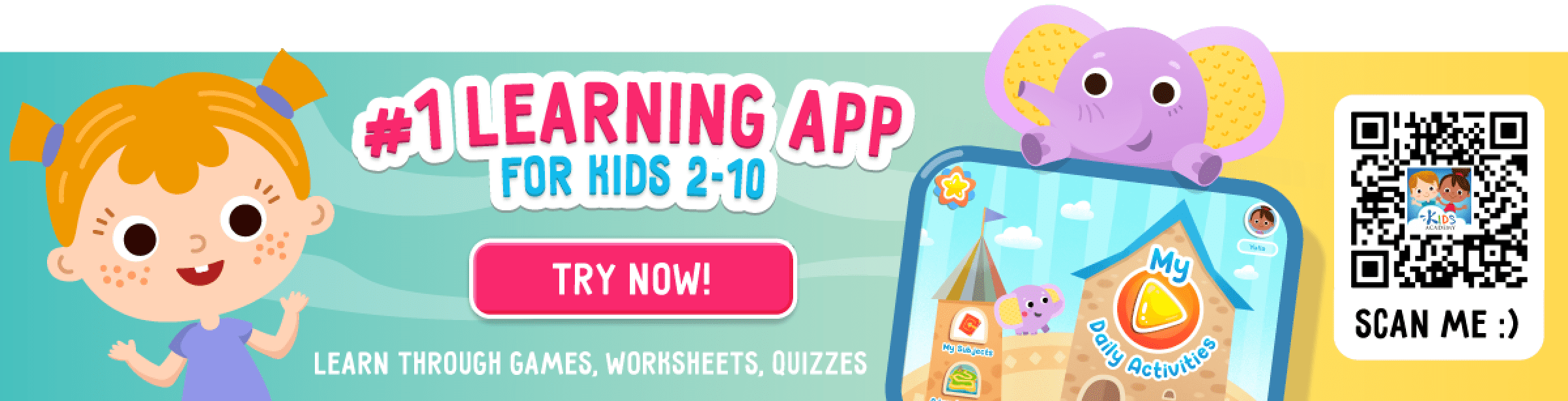# Subtraction Worksheets for Grade 1

25 results

This collection of Grade 1 printable worksheets offers a wide range of tasks to suit your child's learning needs.

Bright pictures and motivating contexts help young students to get engaged in using subtraction to solve equations and word problems and also develop their critical thinking.

Browse our worksheets, print or complete them online to keep young minds interested in math for the years to come!

• Subtraction
Favorites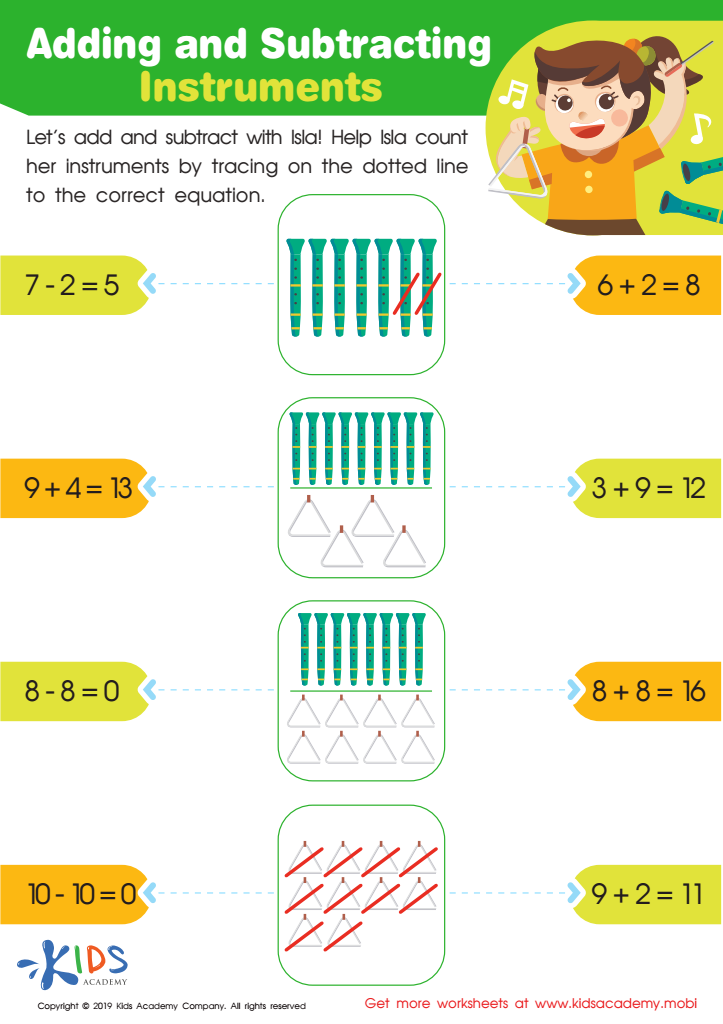Worksheet
This fun worksheet lets children help their friend Isla as she counts the musical instruments …
Subtraction
Worksheet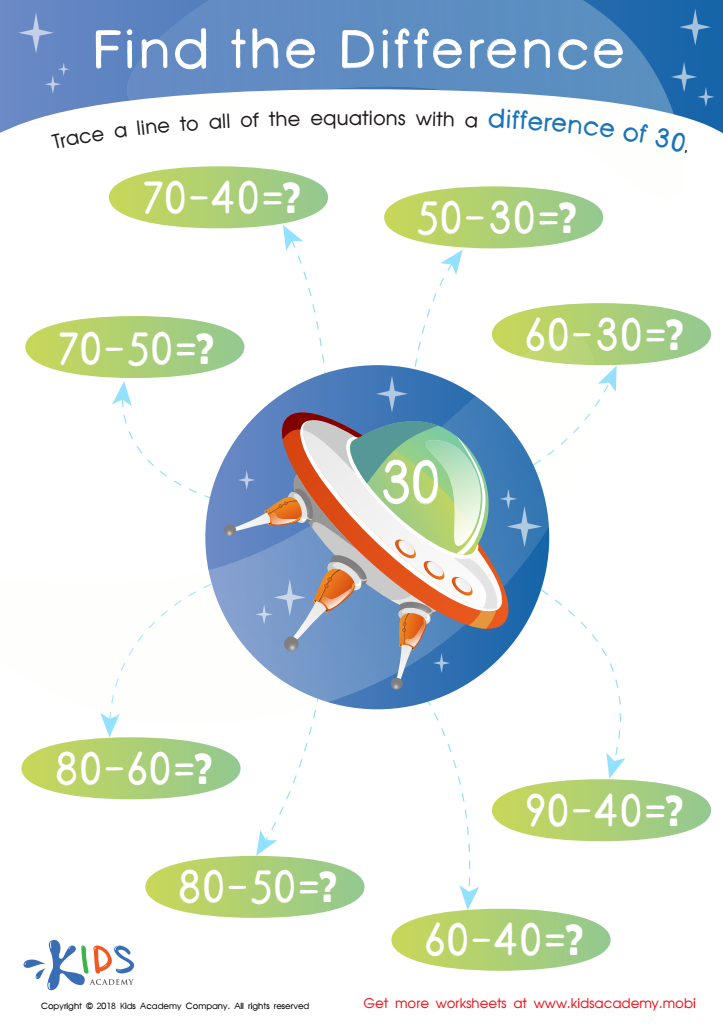Find The Difference Worksheet
Worksheet
Find The Difference Worksheet
Little kindergarteners must be able to correctly solve subtraction problems and arrive at the correct …
Subtraction
Worksheet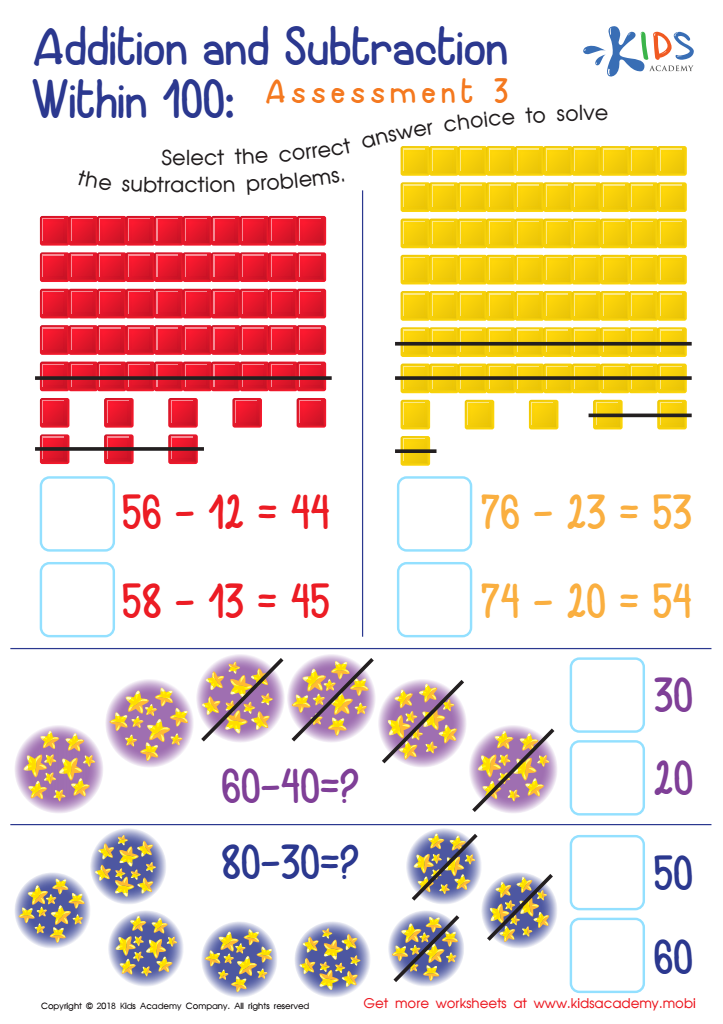Addition and Subtraction Within 1: Assessment 3 Worksheet
Worksheet
Addition and Subtraction Within 1: Assessment 3 Worksheet
Counting base ten blocks and subtraction skills are the two things your kids will have …
Subtraction
Worksheet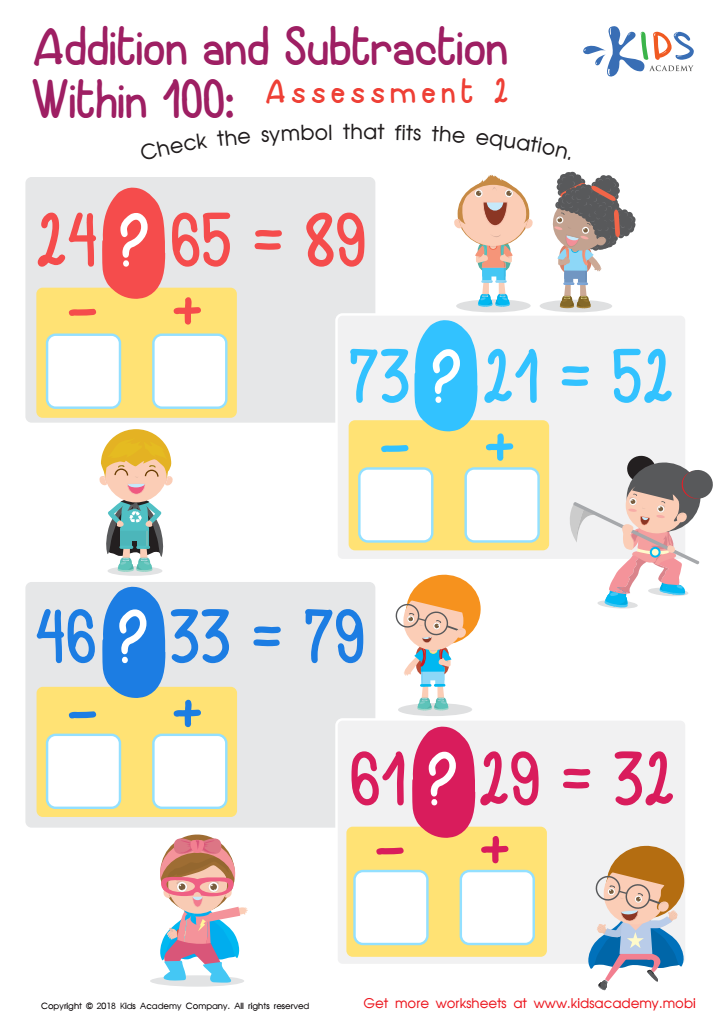Addition and Subtraction Within 1: Assessment 2 Worksheet
Worksheet
Addition and Subtraction Within 1: Assessment 2 Worksheet
If you are teaching your students addition and subtraction in class now, this worksheet will …
Subtraction
Worksheet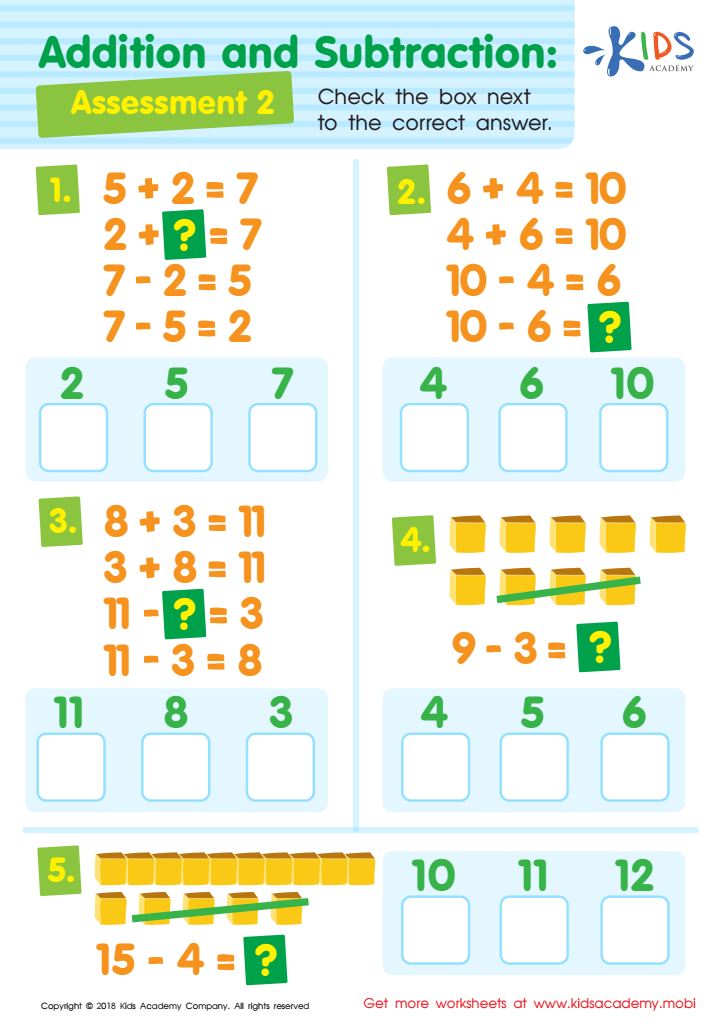Addition and Subtraction Assessment 2 Worksheet
Worksheet
Addition and Subtraction Assessment 2 Worksheet
As your child progresses in age and class, the mathematical problems they have to solve …
Subtraction
Worksheet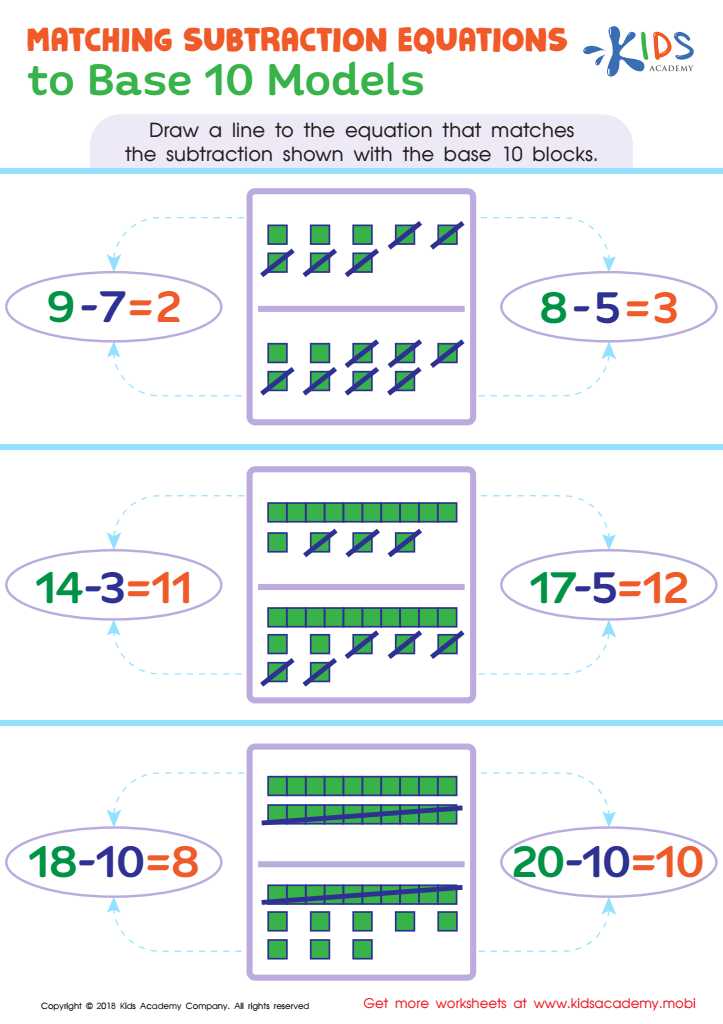Matching Subtraction Equations To Base 10 Models Worksheet
Worksheet
Matching Subtraction Equations To Base 10 Models Worksheet
Subtraction
Worksheet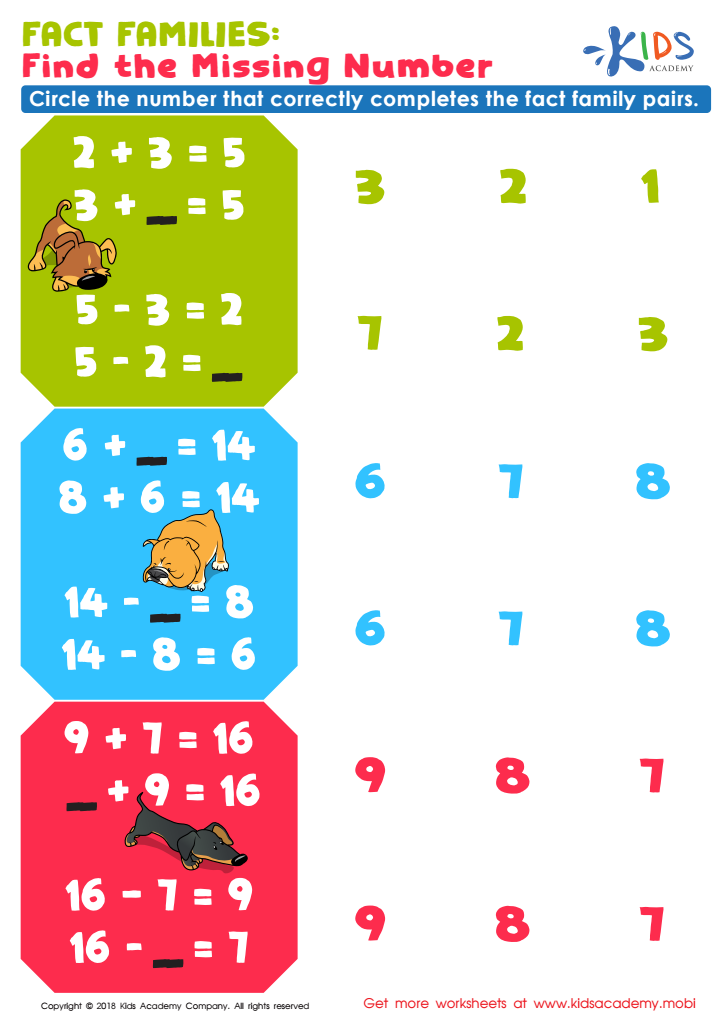Fact Families: Find Missing Number Worksheet
Worksheet
Fact Families: Find Missing Number Worksheet
How good are your students now with adding and subtracting numbers correctly? You can use …
Subtraction
Worksheet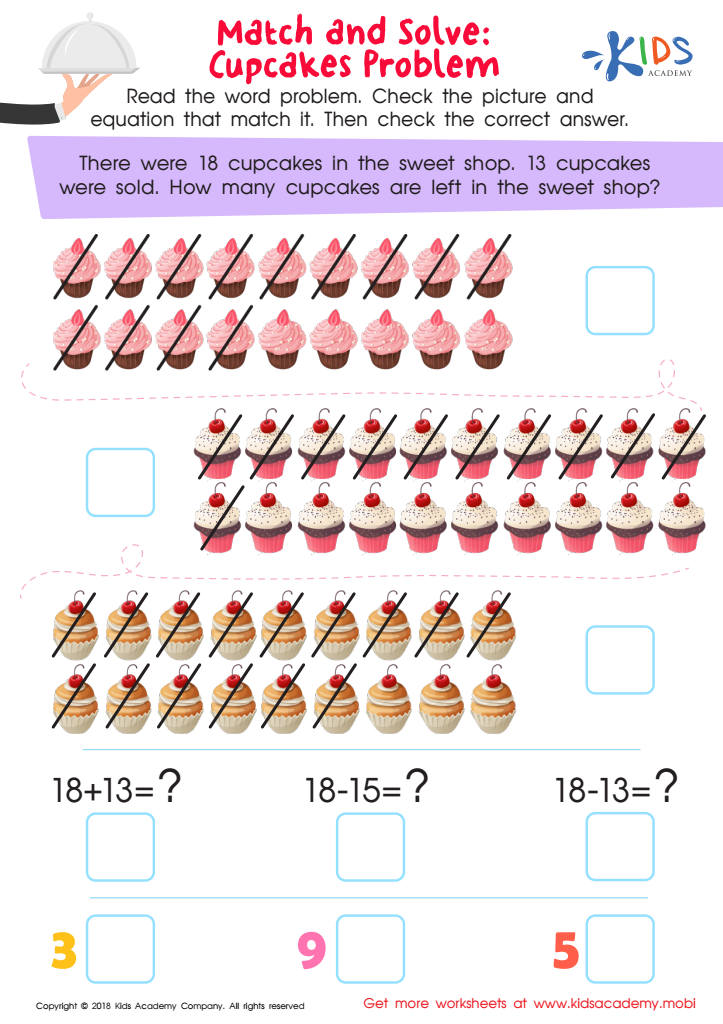Match and Solve: Cupcakes Problem Worksheet
Worksheet
Match and Solve: Cupcakes Problem Worksheet
This colorful math worksheet will tickle your child’s sweet tooth, all while helping him or …
Subtraction
Worksheet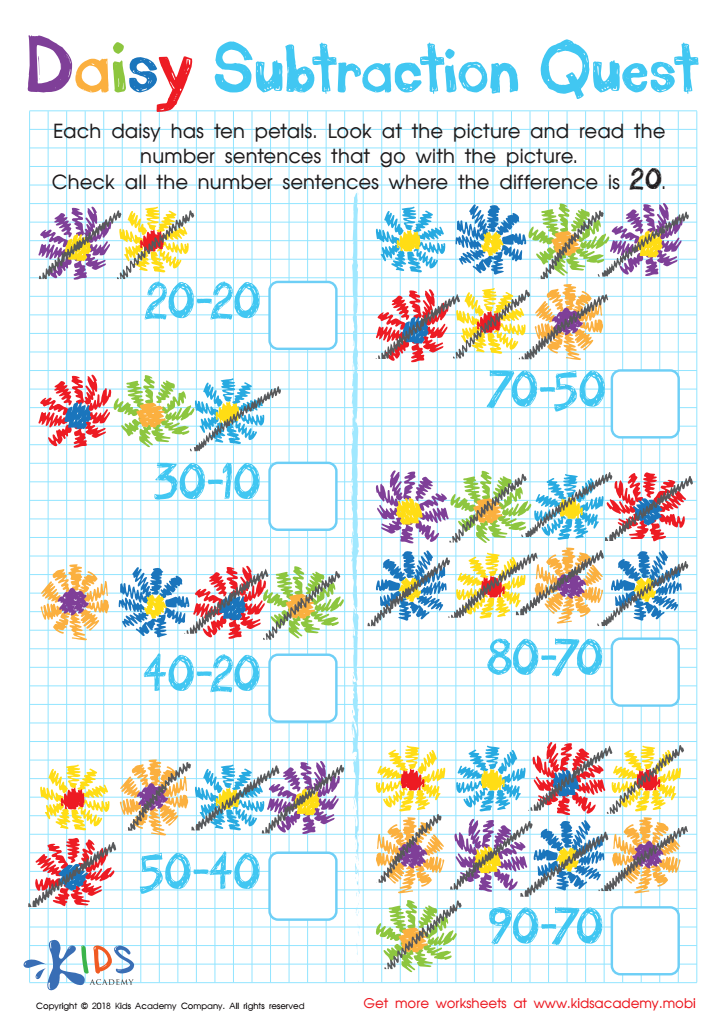Daisy Subtraction Quest Worksheet
Worksheet
Daisy Subtraction Quest Worksheet
Ohh-la-la! These gorgeous daisies aren't your typical flowers! No, they're each designed with ten pretty …
Subtraction
Worksheet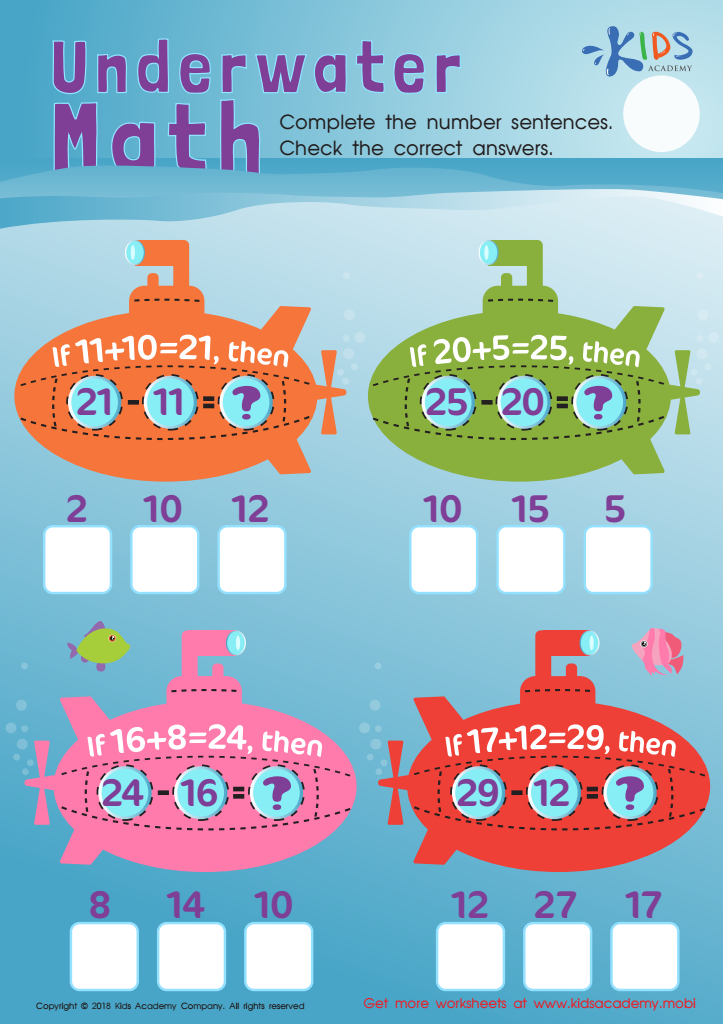Addition and Subtraction: Underwater Math Worksheet
Worksheet
Addition and Subtraction: Underwater Math Worksheet
Let your little mathematicians take an undersea adventure as they work to complete the number …
Subtraction
Worksheet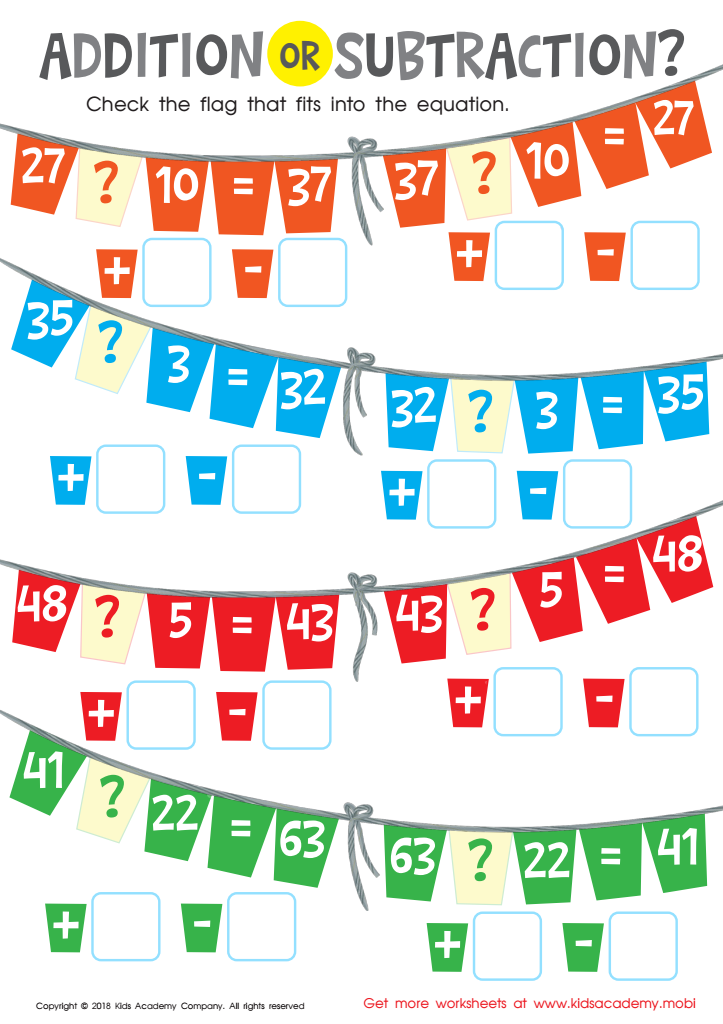Worksheet
Watch your little math whiz's number reasoning and number sense soar with these brightly colored …
Subtraction
Worksheet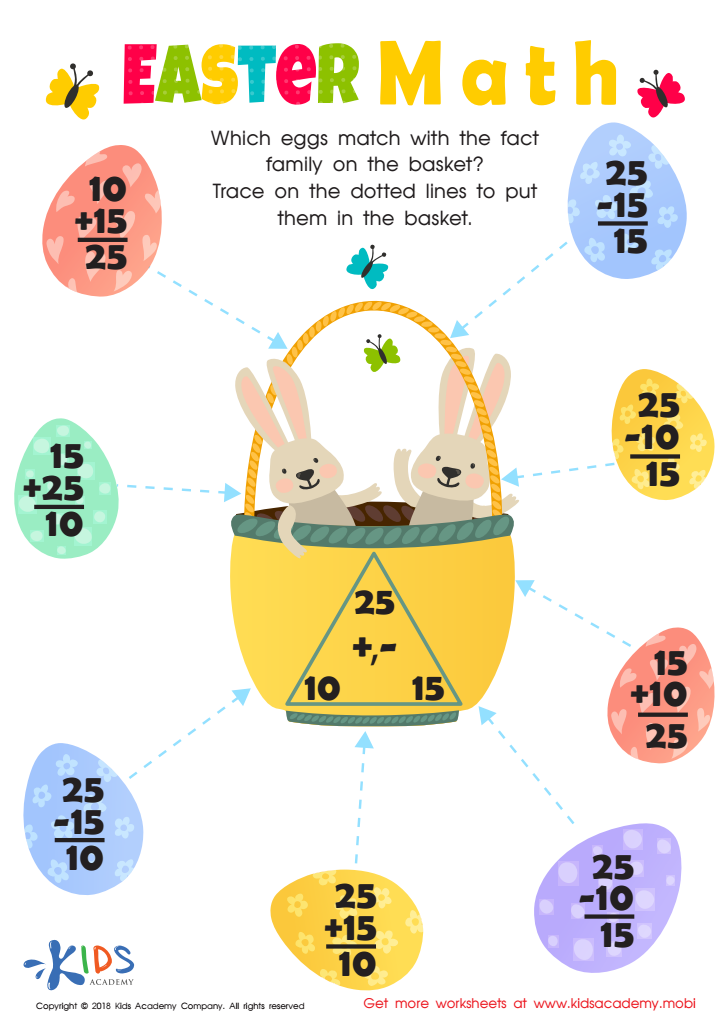Fact Families: Easter Math Worksheet
Worksheet
Fact Families: Easter Math Worksheet
Your little bunnies will adore this fun and colorful PDF that brings Easter math to …
Subtraction
Worksheet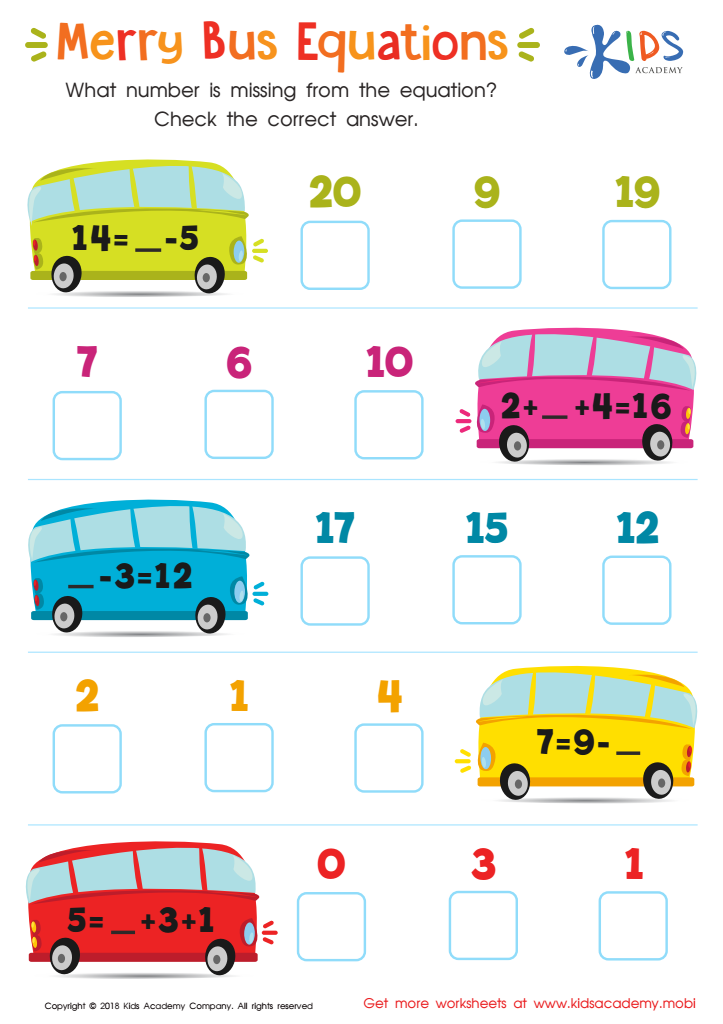Merry Bus Equations Worksheet
Worksheet
Merry Bus Equations Worksheet
Give your child the practice needed to be successful in elementary math! This colorful worksheet …
Subtraction
Worksheet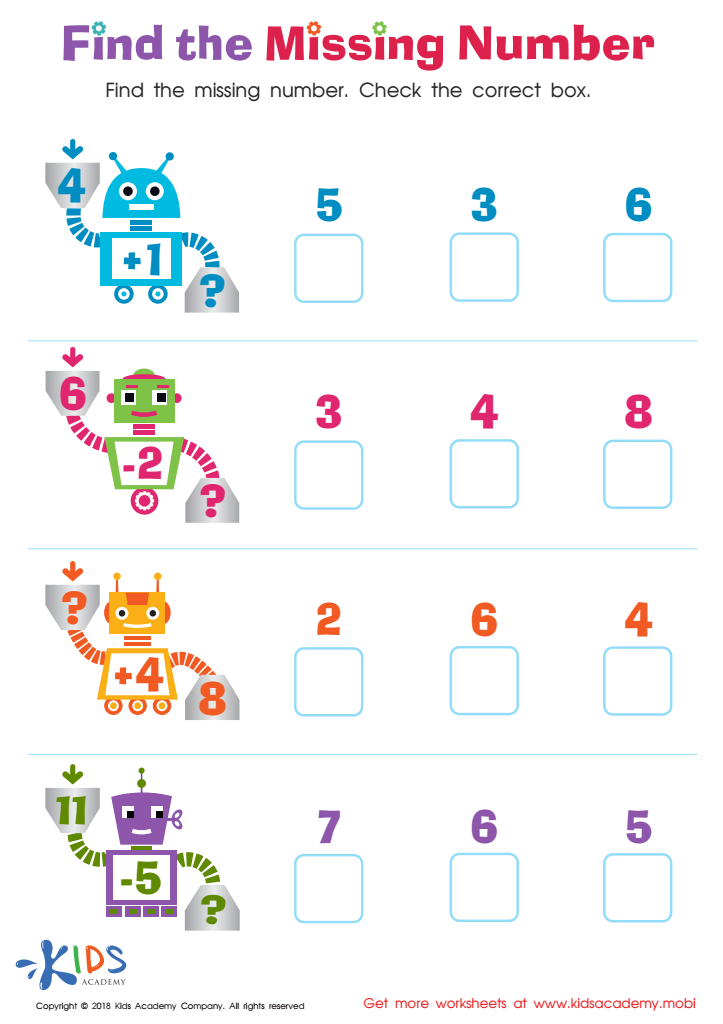Find the Missing Number Worksheet
Worksheet
Find the Missing Number Worksheet
Both repetition and frequent practice are critical for success when it comes to learning anything, …
Subtraction
Worksheet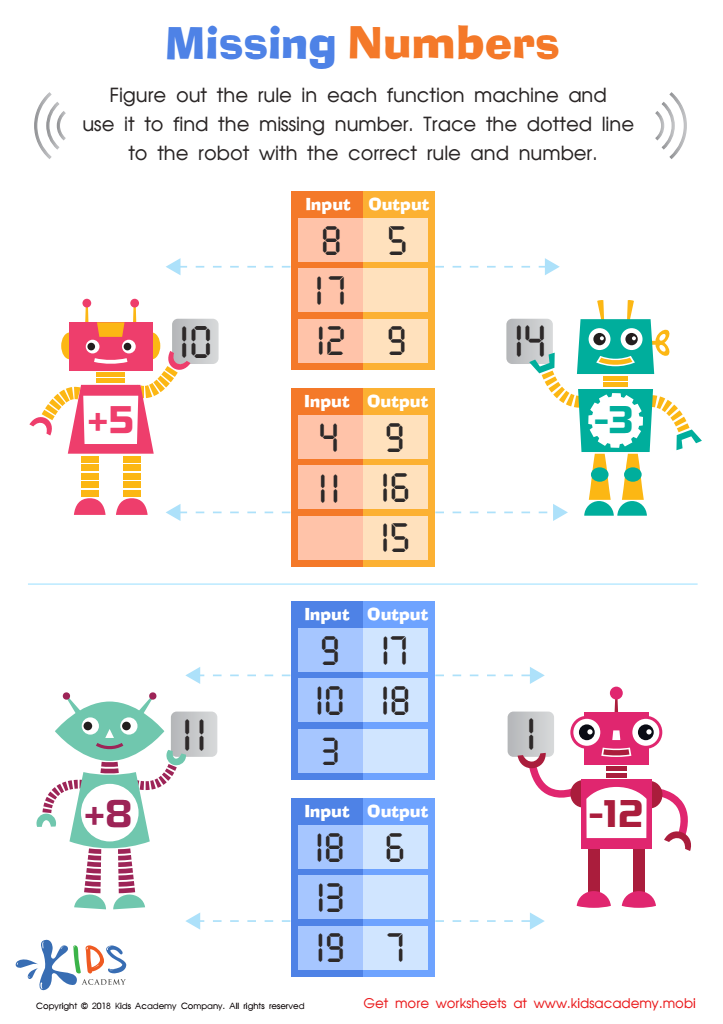Missing Numbers Worksheet
Worksheet
Missing Numbers Worksheet
Solving for missing numbers is a critical pre-algebra skill that even elementary students can learn! …
Subtraction
Worksheet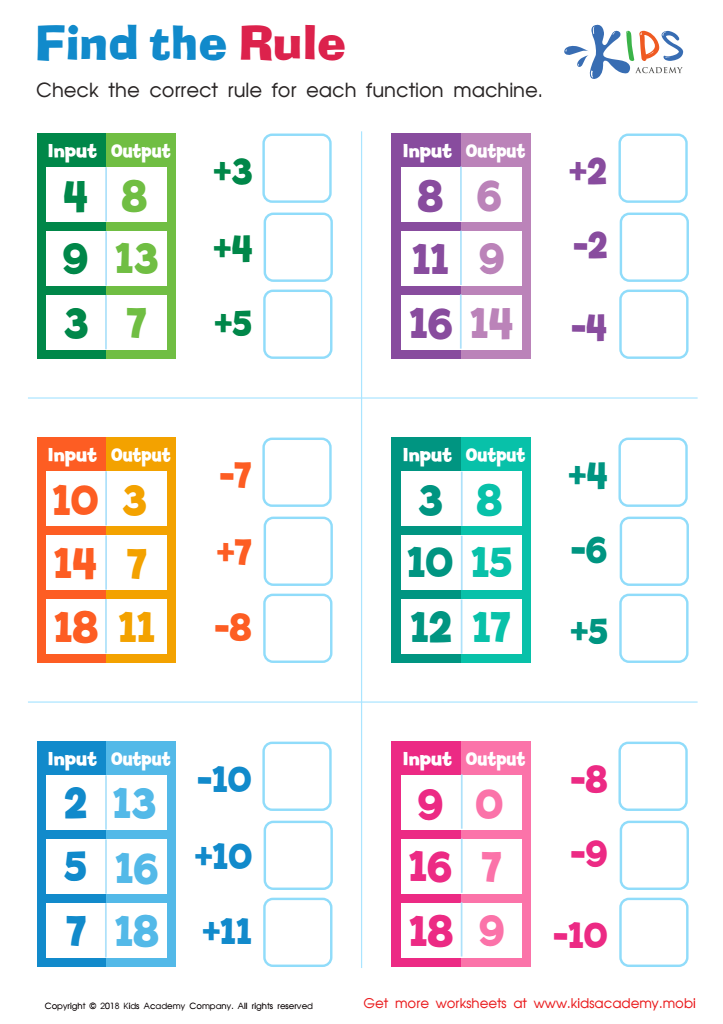Find the Rule Worksheet
Worksheet
Find the Rule Worksheet
In elementary math, a function machine is a diagram that contains an input that applies …
Subtraction
Worksheet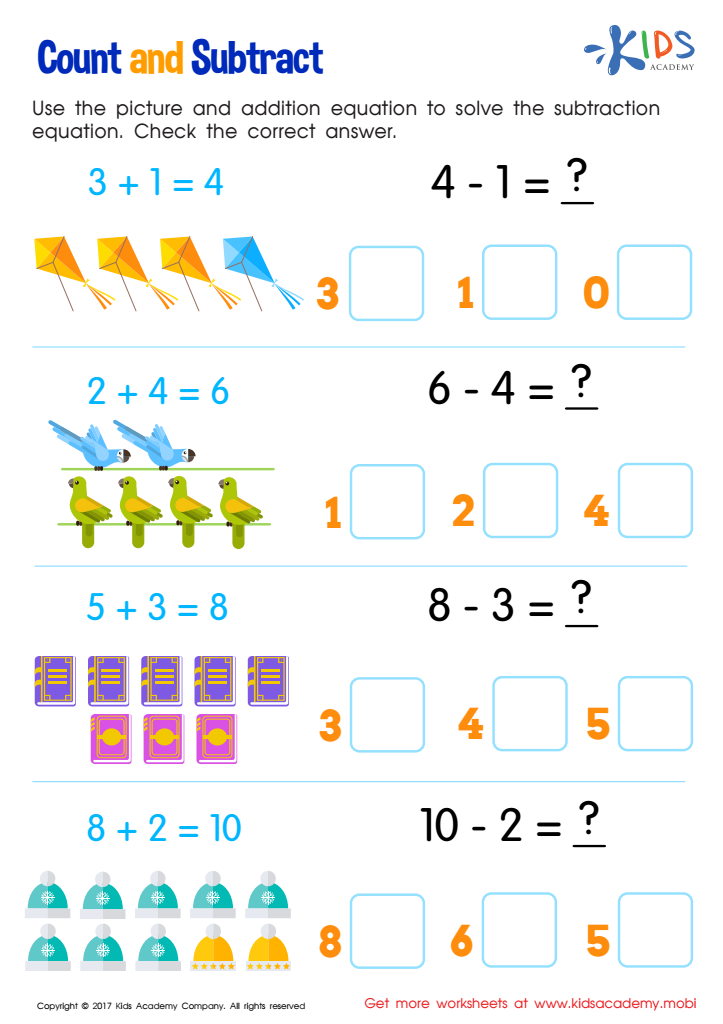Count and Subtract Worksheet
Worksheet
Count and Subtract Worksheet
Colors bring this worksheet to life for your child. The exercise uses pictures as well …
Subtraction
Worksheet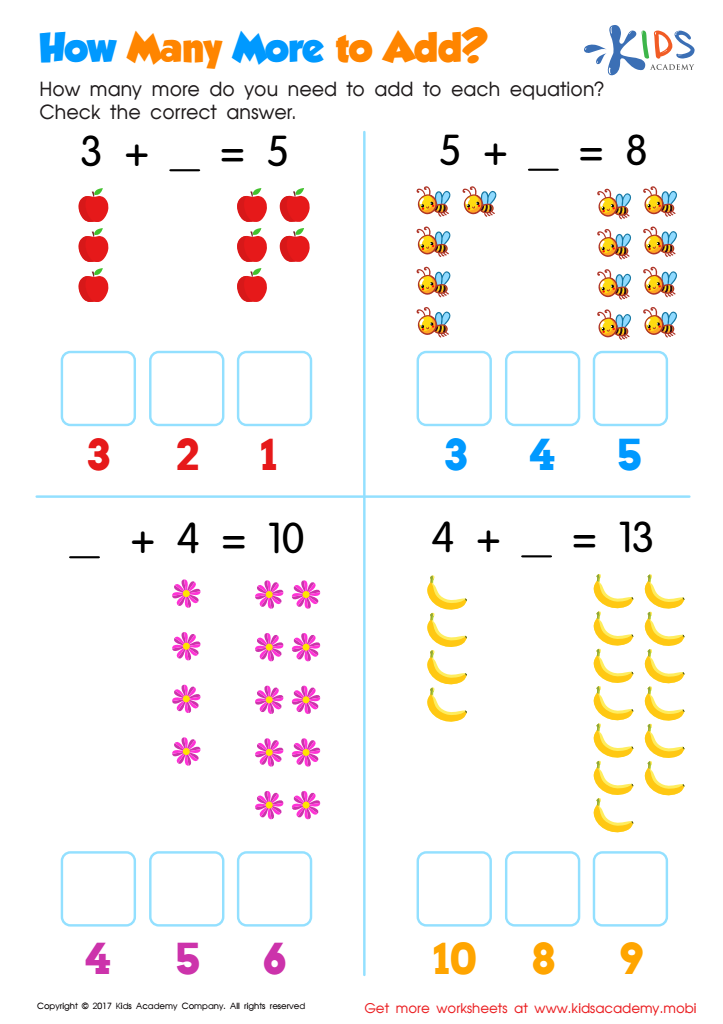How Many More to Add Worksheet
Worksheet
How Many More to Add Worksheet
Sharpen your child’s addition and subtraction skills with the pdf worksheet. How many more apples, …
Subtraction
Worksheet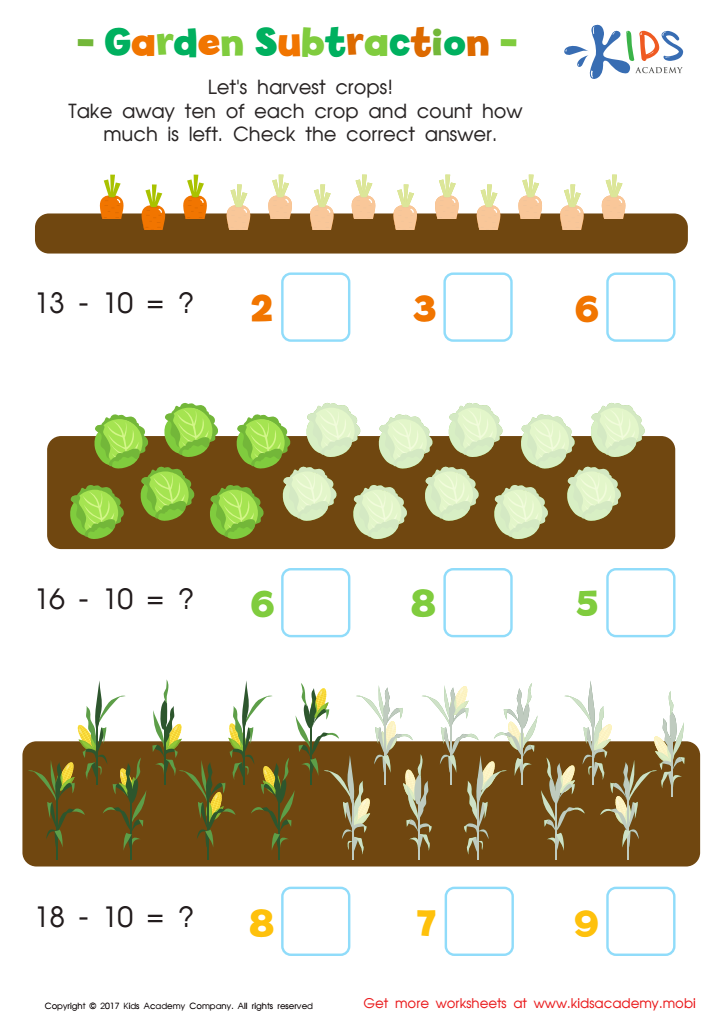Garden Subtraction Worksheet
Worksheet
Garden Subtraction Worksheet
Number sentences are difficult to solve if students don’t understand the underlying concept behind the …
Subtraction
Worksheet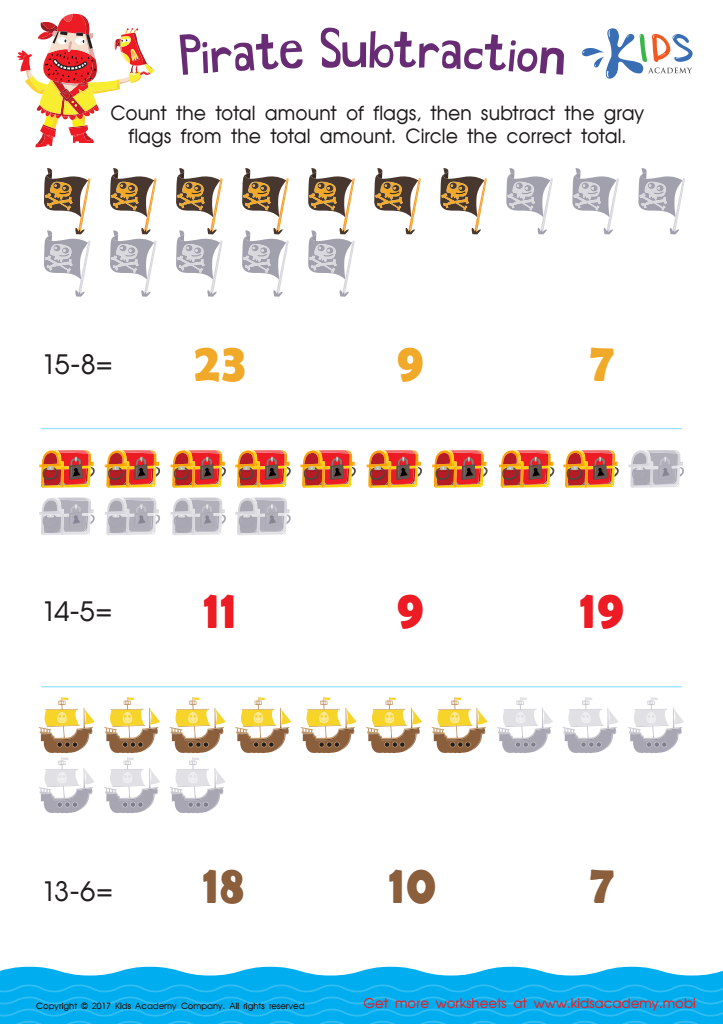Pirate Subtraction Substraction Worksheet
Worksheet
Pirate Subtraction Substraction Worksheet
Ahoy, matey! Let’s learn to subtract the pirate way, with this fun-filled math worksheet: pirate …
Subtraction
Worksheet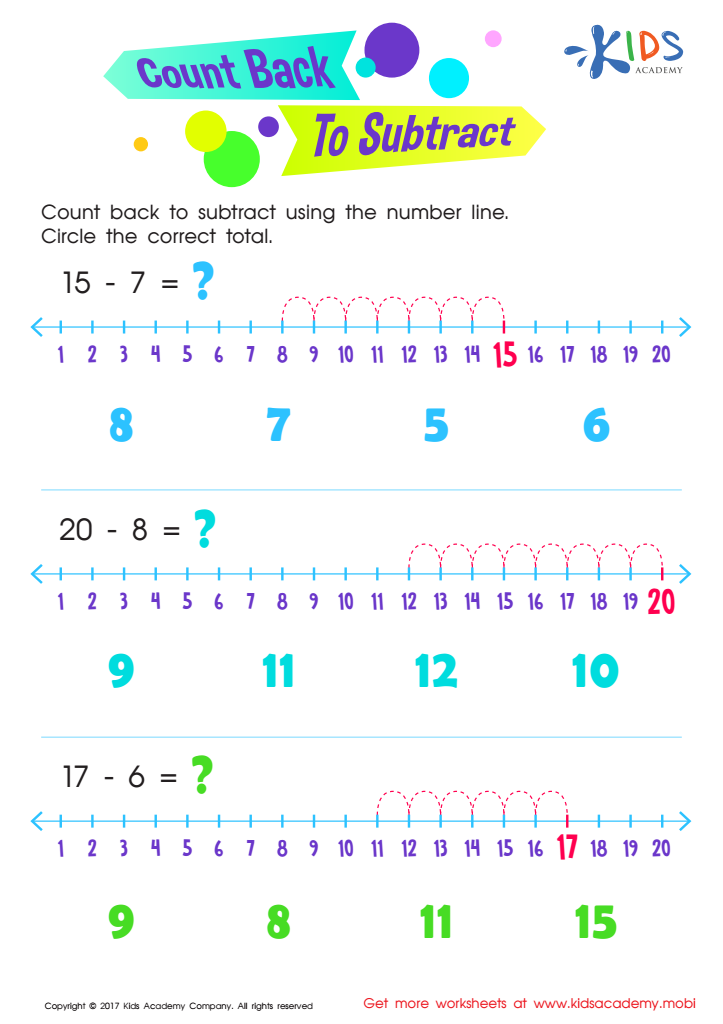Count Back to Subtract Substraction Worksheet
Worksheet
Count Back to Subtract Substraction Worksheet
If you’re looking for yet another strategy to teach your child to subtract, this Count …
Subtraction
Worksheet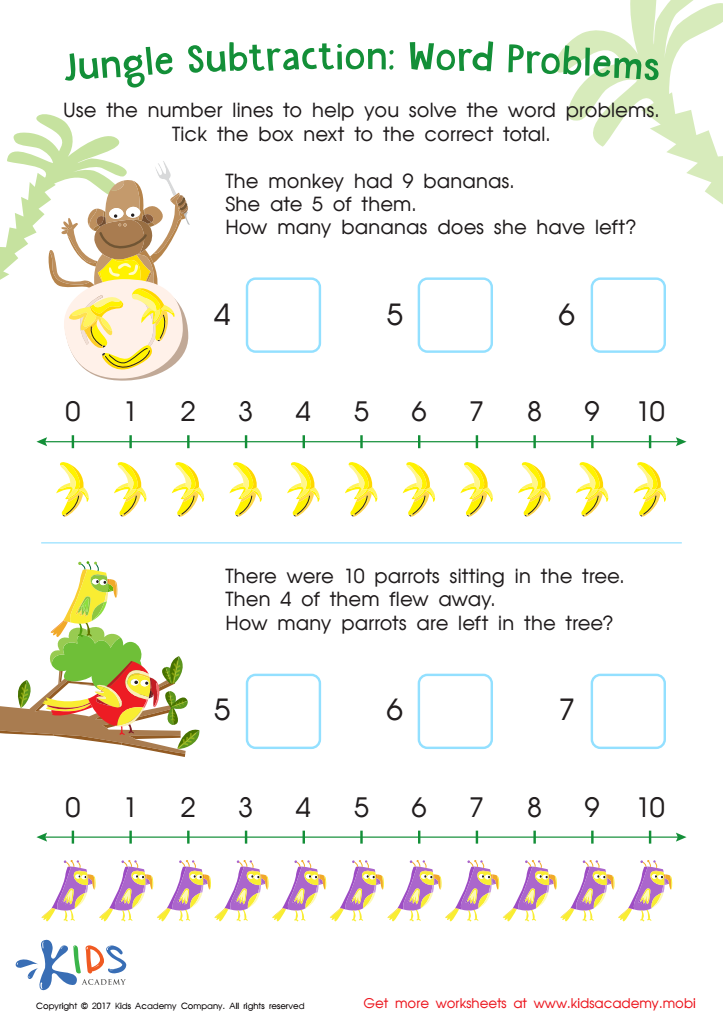Jungle Subtraction Word Problems Substraction Worksheet
Worksheet
Jungle Subtraction Word Problems Substraction Worksheet
Your child will go bananas over this amusing jungle-themed worksheet: subtraction word problems! Read through …
Subtraction
Worksheet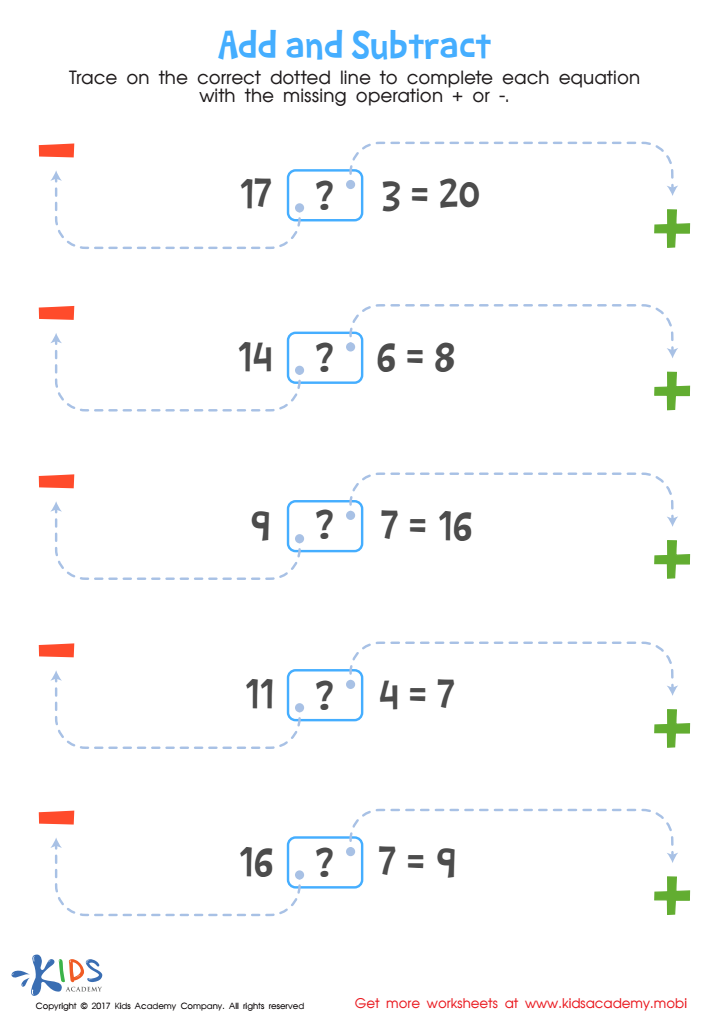Worksheet
As kids learn the basics of math, it’s important to expose them to equations early …
Subtraction
Worksheet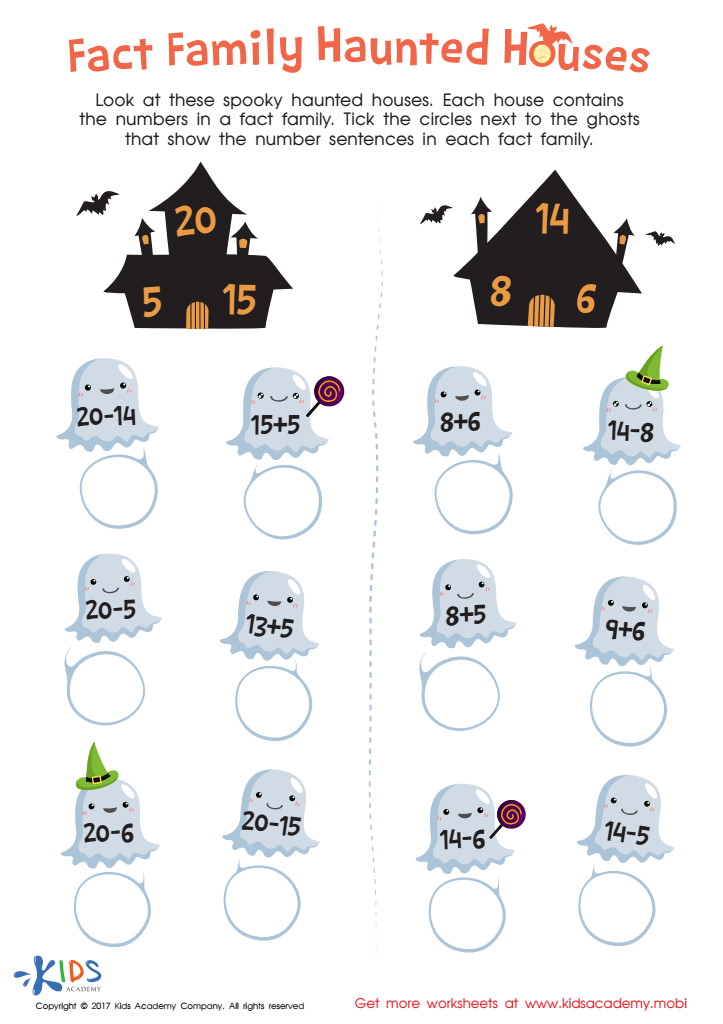Family Haunted Houses Printable
Worksheet
Family Haunted Houses Printable
Kids need a variety of ways to practice math skills and concepts. If your child …
Subtraction
Worksheet

Note: You will not be billed until your free trial has ended and can cancel at any time. No strings attached.

Daughter's favorite
Still a top favorite for our 7 year old. She loves earning her stars as she learns to trace letters and numbers. Coloring and the sounds are engaging and are on target for her age group and being Pre K.
Surviving5Kids
Great app!
This app is wonderful for my 7 years old son! :) It focuses on basic reading, writing, and math skills. The reward system is great and consistent!
Lilbittygarza
Great for kids
Our 7 years old daughter loves reading activities and every off-line game Kids Academy offers. This is a very good app, I highly recommend it!
TonyThan
So engaging
We love how we can focus on math with this app. It is engaging and we love how we are able to adjust and tailor the levels according to our son’s knowledge and skill levels!
Momma Anna
Such a great app!
This was a really fun way to get my kids to practice their math. I usually struggle to get them into it and Kids Academy makes it easy.
Fernanndas Stoun
Great app
Wow! This app is really great. For the first time my kids actually enjoy learning math and reading. The games on Kids Academy are educational and really fun.
Kimberlyxo